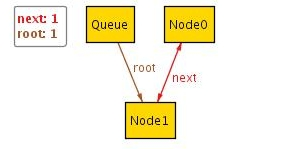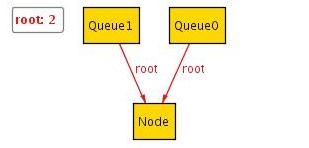A Guide to Alloy
 Home Introduction Tutorial Getting Started Statics I Statics II Dynamics I Dynamics II Alternatives to Alloy Test Yourself! Glossary FAQs Final Thoughts
Statics II
To address the issue of nodes that do not belong to any queues, we add another fact:
```	fact allNodesBelongToSomeQueue {
all n:Node | some q:Queue | n in q.root.*next
}
```
all and some behave exactly like the forall and exists quantifiers in predicate logic. The .* operator represents the reflexive transitive closure: It returns the set consisting of all elements
```	q.root,
q.root.next,
q.root.next.next,
...
```
If we press Execute, Alloy tells us that it found an instance. However, when we ask it to visualize the instance, it tells us that every atom is hidden. In order to get the next solution, we click Next at the top. Browsing through the instances, we find that one of them contains a cycle:
 ``` module examples/tutorial/Queue sig Queue { root: Node } sig Node { next: lone Node } fact nextNotReflexive { no n:Node | n = n.next } fact allNodesBelongToSomeQueue { all n:Node | some q:Queue | n in q.root.*next } pred show() {} run show for 2 ```In order to fix our model we add another fact:
```	fact nextNotCyclic { no n:Node | n in n.^next }
```
In contrast to the .* operator, .^ represents the non-reflexive transitive closure which returns the set consisting of all elements:
```	n.next,
n.next.next,
n.next.next.next,
...
```
(Note that this set does not include n itself).

Executing, visualizing and browsing through a few instances, we spot another error:
 ``` module examples/tutorial/Queue sig Queue { root: Node } sig Node { next: lone Node } fact nextNotReflexive { no n:Node | n = n.next } fact allNodesBelongToSomeQueue { all n:Node | some q:Queue | n in q.root.*next } fact nextNotCyclic { no n:Node | n in n.^next } pred show() {} run show for 2 ```The problem here is that Node0 belongs to two different queues. This is because allNodesBelongToSomeQueue constrains a Node to belong to some Queue while it should actually belong to exactly one. To fix this we modify the fact:
```	fact allNodesBelongToOneQueue {
all n:Node | one q:Queue | n in q.root.*next
}
```
Browsing through the instances for this version of the model, Alloy soon tells us that 'there are no more satisfying instances'. All the solutions found within the scope provided seem to be correct. To gain more confidence, we increase the scope further:
```	run show for 3
```
Still, we cannot find any instances that clash with our definition of a linked list and conclude that this seems to be a good model for a linked list implementation of a queue. However, note that this does not prove that our model is correct! We can only guarantee that it corresponds with our definition for at most three Queues and three Nodes. As Alloy only ever searches a finite scope, it never allows you to prove that your model is correct. However, you can gain a fair amount of confidence that the main errors have been eliminated.

Now that we have seen how to model the static aspects of a system, we will move on to modelling dynamic behaviour and adding operations. For this, we will consider a different example.